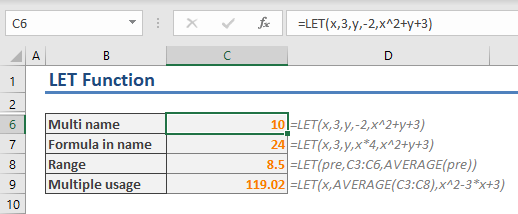The LET function can define names within the scope of a formula. This essentially offers better readability and help with calculation performance. In this guide, we are going to show you how to use the LET function and go over some tips and error handling methods.

## Supported versions

*At the time of writing this article, this is a beta feature.

## Syntax

LET(name1, name_value1, calculation_or_name2, [name_value2, calculation_or_name3...])

## Arguments

 name1 The first in-formula name. The name must satisfy named range naming convention in Excel. name_value1 The value of the name1. This can be a static value, a reference, or another formula. calculation_or_name2 One of the following: ·         If this is the last argument, it should be the calculation uses the names defined within the function. ·         The second in-formula name. [name_value2, calculation_or_name3...] Optional. The value of the name2. Otherwise, you can omit this value.

## LET Function Examples

### Simple Usage

The LET function gives you the ability to add names like variables in programming, and use them as much as you want in the formula.

The first arguments should be name and name_value pairs. You can define up to 126 pairs. The last argument should always be the calculation which uses the defined names.

For example, the following formula defines the name “x” and assigns 3 to it. The x will be used in the calculation defined in the last argument, x*2+1.

=LET(x,3,x*2+1)Here are more examples for the LET function. As shown in the next example, with this formula you can,

• use a defined name in another name’s expression
• choose to use a reference as a name_value
• use the defined named range multiple times in the calculationLet's say you want to label your departments based on employee’s average performance scores. An expression like below can be used for this.

• if the average score is below 3 then it is “below average”.
• if the average score is below 4 then it is “average”.
• the rest is “above average”.

If the average formula is like the following:

AVERAGEIF(Employee[Department],M2#,Employee[Performance Score])

The expression should be:

=IF(AVERAGEIF(Employee[Department],M2#,Employee[Performance Score])<=3,"Below Average",IF(AVERAGEIF(Employee[Department],M2#,Employee[Performance Score])<=4,"Average","Above Average"))

This a relatively simple formula, but it's hard to read as the average expression is used twice.If you are wondering what M2# means, this is a dynamic array reference which refer the cells starting from M2 cell. Please see the Spill Range Operator article to learn more about this feature.

Here is how the formula would be using the LET function instead:

=LET(AvgScore,AVERAGEIF(Employee[Department],M2#,Employee[Performance Score]),IF(AvgScore<=3,"Below Average",IF(AvgScore<=4,"Average","Above Average")))

The average expression is defined to a name called “AvgScore”. Once defined, you can use the name in the calculation, instead of the full formula.## Tips and Remarks

• Use the LET function if you need to use the same expression multiple times in a formula. If you define the expression by a name in the LET function, Excel calculates it only once.
• Note that you may not have access to this formula even if you are an Office Insider subscriber.
• When creating names using the LET function, you must follow the same rules for creating named ranges. Avoid using references as names, otherwise you will get an error message. For example, “x1” gives an error because it's a cell reference.